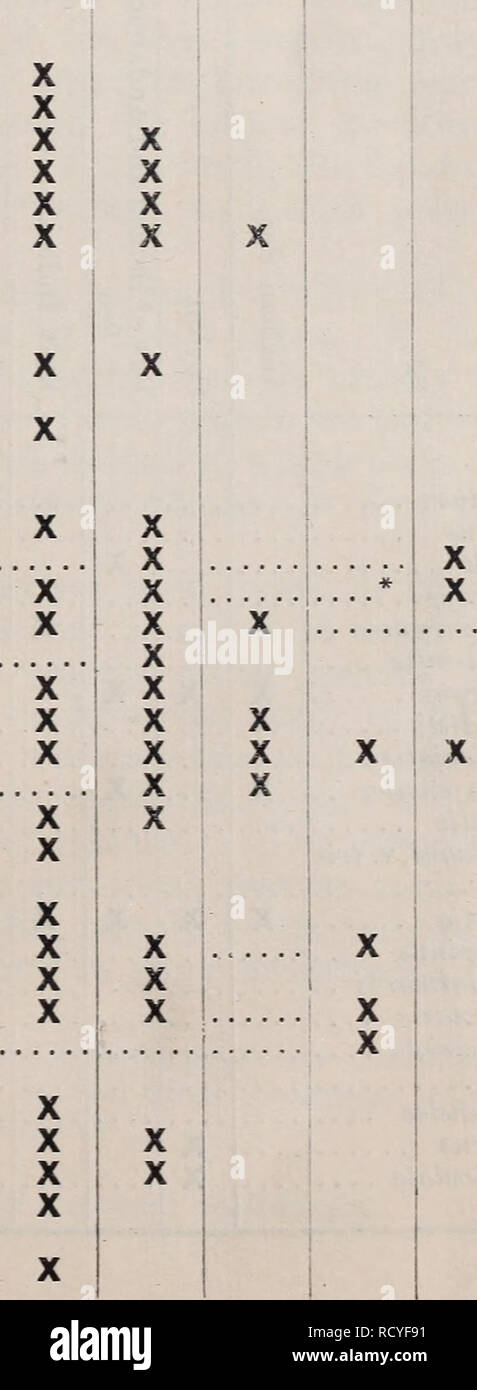# . Decapoda natantia of the coasts of Ireland. Decapoda (Crustacea). AND IRISH DECAPODA NATANTIA. 1 2 3 4 5 6 7 8 9 10 11 12 13 14 15 16 17 18 19 20 21 22 23 24 25 26 27 28 29 30 31 32 33 34 35 36 37 38 39 40 41 42 43 44 45 46 47 48 49 50 51 52 53 54 o o -*3 'â *3 o o u (H &lt; &lt; &lt;*- J &gt;Â» ce d ^ ^ u (1 cS o o O o O O a u a a eg PQ ID ed ^ s eft fcf 9i a c8 C o m â¢So â¢^ &lt;11 Oi a a 09 ^ in a et cn 5 73 -2 0) in d c3 ^ X X X X X X X X X X X X X X X X X X X X X X X X X X X X X X X X X X X X X X X X X X X X X X X X X X X X X X X X X X X X X X X X X X X X X X X X X X X X X X X X X X X## Image details

Contributor:

Paul Fearn / Alamy Stock Photo

Image ID:

RCYF91

File size:

Releases:

Model - no | Property - noDo I need a release?

Dimensions:

958 x 2608 px | 8.1 x 22.1 cm | 3.2 x 8.7 inches | 300dpi

This image is a public domain image, which means either that copyright has expired in the image or the copyright holder has waived their copyright. Alamy charges you a fee for access to the high resolution copy of the image.

This image could have imperfections as it’s either historical or reportage.

. Decapoda natantia of the coasts of Ireland. Decapoda (Crustacea). AND IRISH DECAPODA NATANTIA. 1 2 3 4 5 6 7 8 9 10 11 12 13 14 15 16 17 18 19 20 21 22 23 24 25 26 27 28 29 30 31 32 33 34 35 36 37 38 39 40 41 42 43 44 45 46 47 48 49 50 51 52 53 54 o o -*3 'â *3 o o u (H &lt; &lt; &lt;*- J &gt;Â» ce d ^ ^ u (1 cS o o O o O O a u a a eg PQ ID ed ^ s eft fcf 9i a c8 C o m â¢So â¢^ &lt;11 Oi a a 09 ^ in a et cn 5 73 -2 0) in d c3 ^ X X X X X X X X X X X X X X X X X X X X X X X X X X X X X X X X X X X X X X X X X X X X X X X X X X X X X X X X X X X X X X X X X X X X X X X X X X X X X X X X X X X X X X X X X X X X X. X X * Known from Madeira.. Please note that these images are extracted from scanned page images that may have been digitally enhanced for readability - coloration and appearance of these illustrations may not perfectly resemble the original work.. Kemp, Stanley. Dublin, Alexander Thom &amp; Co. , 1910

Save up to 30% with our image packs